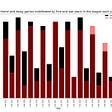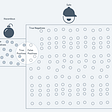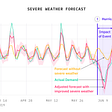# Exploratory Data Analysis on Covid-19 dataset

As part of my course project on Data Analysis with Python, I had to first find a real-world dataset and perform an exploratory data analysis on it. Without much thought, I decided to work on the most trending topic in today’s world — Covid-19. I downloaded the latest dataset on Covid-19 from https://ourworldindata.org/coronavirus-source-data which gave a complete list of information for all the countries starting from February 24, 2020. Similarly, I downloaded another dataset from https://www.kaggle.com/fernandol/countries-of-the-world. This dataset contained other basic information of the countries(not covid related). I wanted to merge certain columns from both these datasets for my analysis.

For this project, I have used Pandas, matplotlib and Seaborn.

Pandas is a python library that contains data structures and data manipulation tools. It is used for working with tabular or heterogeneous data. Similarly, Matplotlib is a python plotting library which is designed for basic plotting(bars, lines, scatter plots, etc). Seaborn provides a variety of visualization patterns and uses fewer syntax. It is used in statistics visualization to summarize the data in visualizations or to show the distribution.

`#Importing the required librariesimport numpy as npimport pandas as pdimport matplotlibimport matplotlib.pyplot as pltimport seaborn as sns%matplotlib inline`

## Data Preparation and Cleaning:

Let me first load the required datasets into a panda dataframe:

`#Loading data from the first csv file into a dataframecovid_data_df = pd.read_csv('./covid-data.csv')covid_data_df`
`#Loading data from the next csv file into another dataframecountries_data_df = pd.read_csv('./countries-of-the-world.csv')countries_data_df`

This is followed by cleaning the dataset. This process involves handling missing and invalid data, grouping by certain columns, selecting required columns, and finally merging the datasets to get a finalized dataframe.

For the first Dataset:

`covid_data_df = covid_data_df.rename(columns = {'location' :'Country'}, inplace = False) #renaming the column 'location' to 'Country'#creating a dataframe with only the columns I requirenew_covid_data_df = covid_data_df[['Country','date','continent', 'population', 'gdp_per_capita', 'life_expectancy', 'male_smokers', 'female_smokers' ]]#Grouping all values by the 'Country' column, choosing the first value from its multiple rows and then reseting the indexnew_covid_data_df= new_covid_data_df.groupby('Country').first().reset_index()#Removing the rows that have na/none in 'continent' columnnew_covid_data_df=new_covid_data_df[new_covid_data_df['continent'].notna()].reset_index()#dropping the index columnnew_covid_data_df= new_covid_data_df.drop('index', axis=1)#dropping the date columnnew_covid_data_df= new_covid_data_df.drop('date', axis=1)#removing spaces at the beginning and at the endnew_covid_data_df['Country'] = new_covid_data_df['Country'].str.strip()`

## The first dataframe:

`new_covid_data_df`

## For the second dataset:

`#creating a dataframe with only the required columnsnew_countries_data_df = countries_data_df[['Country', 'Region', 'Net migration', 'Literacy (%)']]new_countries_data_df['Country'] = new_countries_data_df['Country'].str.strip()<ipython-input-41-616a771263d6>:1: SettingWithCopyWarning: A value is trying to be set on a copy of a slice from a DataFrame.Try using .loc[row_indexer,col_indexer] = value insteadSee the caveats in the documentation: https://pandas.pydata.org/pandas-docs/stable/user_guide/indexing.html#returning-a-view-versus-a-copy  new_countries_data_df['Country'] = new_countries_data_df['Country'].str.strip()`

## The second dataframe:

`new_countries_data_df`

Looks like the second dataframe does not need any further cleaning, so moving on to the next step.

I’m now creating a new dataframe to calculate the cumulative number for all the countries which I will be using later. We can see that the initial dataset of covid contains the information about the countries on each date starting from 22nd Feb 2020 to 9th June 2021, where the number on 9th June gives the latest data. Let’s call it the third dataframe.

`total_covid_data_df = covid_data_df.groupby('Country').last()`

## Data cleaning:

`#removing rows that have 0 life expectancytotal_covid_data_df = total_covid_data_df[total_covid_data_df['life_expectancy'] !=0].reset_index()#creating a df with only the required columnsnew_total_covid_data_df = total_covid_data_df[['Country','hospital_beds_per_thousand','total_cases', 'total_deaths', 'total_tests']]`

## The third dataframe:

`new_total_covid_data_df`

Now, I merge the three dataframes to get my finalized dataframe:

`#merging first and second dfs:first_second = new_covid_data_df.merge(new_countries_data_df, on='Country')#merging the result with the third dataframe:my_df = first_second.merge(new_total_covid_data_df, on = 'Country')`

## My finalized dataframe:

`my_df`

Now let's find some details about our dataframe:

`my_df.shape #gives the shape(rows and columns) of the df(189, 14)my_df.info() #gives the summary of the dataframe that includes index dtype and column dtypes, non-null values and memory usage.<class 'pandas.core.frame.DataFrame'>Int64Index: 189 entries, 0 to 188Data columns (total 14 columns): #   Column                      Non-Null Count  Dtype  ---  ------                      --------------  -----   0   Country                     189 non-null    object  1   continent                   189 non-null    object  2   population                  189 non-null    float64 3   gdp_per_capita              170 non-null    float64 4   life_expectancy             187 non-null    float64 5   male_smokers                133 non-null    float64 6   female_smokers              135 non-null    float64 7   Region                      189 non-null    object  8   Net migration               187 non-null    float64 9   Literacy (%)                179 non-null    float64 10  hospital_beds_per_thousand  155 non-null    float64 11  total_cases                 170 non-null    float64 12  total_deaths                165 non-null    float64 13  total_tests                 114 non-null    float64dtypes: float64(11), object(3)memory usage: 19.9+ KBmy_df.describe() #gives the statistical details of the df like percentile, mean, std`

From the above computations, we get a lot of ideas and information about our dataframe. For eg: Our dataframe consists of 189 countries, grouped across 14 different categories. Most of the columns are of float datatype. Similarly, we get the statistics as well. For eg: the mean value of total cases for all the countries is 1007963 and its standard deviation is 3713711. The standard deviation is spread very higher than the mean total cases which means that the changes in the cases are very unpredictable.

Similarly, making some other calculations:

`#Calculating average life expectancy using weighted average formula:prod_le_df = my_df['population']*my_df['life_expectancy']avg_le = prod_le_df.sum()/my_df['population'].sum()print("\n The average life expectancy of the countries is {:.2f} ".format(avg_le))The average life expectancy of the countries is 72.88print("The sum total of all the reported cases upto June 9th, 2021 is {}, out of which {} people have died. The total tests conducted is {}".format(my_df['total_cases'].sum(), my_df['total_deaths'].sum(), my_df['total_tests'].sum()))The sum total of all the reported cases upto June 9th, 2021 is 171353746.0, out of which 3694892.0 people have died. The total tests conducted is 2281432127.0#locating the country with the highest deaths and cases:chc = my_df.loc[my_df['total_cases']==my_df['total_cases'].max(), 'Country'].item()chd = my_df.loc[my_df['total_deaths']==my_df['total_deaths'].max(), 'Country'].item()print("The country with the highest number of cases is {} with {} cases, and that with the highest number of deaths is {} with {} deaths".format(chc, my_df['total_cases'].max(), chd, my_df['total_deaths'].max()))The country with the highest number of cases is United States with 33414025.0 cases, and that with the highest number of deaths is United States with 598764.0 deaths`

Since both the highest cases and the highest deaths are from the United States, it is the most affected country from the pandemic.

## Now, plotting different graphs:

`#configuring some styles first:sns.set_style("darkgrid")matplotlib.rcParams['figure.figsize'] = (11,7)matplotlib.rcParams['font.size'] = 13matplotlib.rcParams['figure.facecolor'] = '#0f0f0f80'my_df_cont = my_df.groupby('continent')[['total_cases', 'total_deaths', 'total_tests', 'population']].sum().reset_index()#plotting a line chart to compare total tests, deaths and cases across the continentsplt.plot(my_df_cont['continent'], my_df_cont['total_cases'])plt.plot(my_df_cont['continent'], my_df_cont['total_deaths'])plt.plot(my_df_cont['continent'], my_df_cont['total_tests'])plt.xlabel('Continents')plt.ylabel('Number in millions')plt.title('Covid-19')plt.legend(['Total Cases', 'Total Deaths', 'Total tests'])<matplotlib.legend.Legend at 0x12136d78>`

From the graph, we can note that the most number of tests have been done in Asia and the least in Oceania. Similarly, the highest number of cases are again from Asia and the lowest from Oceania. But it seems we cannot determine the same for total deaths as the line is somewhat straight. So, let's try to use a barplot to show the total deaths across the continents.

`sns.barplot('continent', 'total_deaths', data= my_df_cont)c:\users\beeka\appdata\local\programs\python\python38-32\lib\site-packages\seaborn\_decorators.py:36: FutureWarning: Pass the following variables as keyword args: x, y. From version 0.12, the only valid positional argument will be `data`, and passing other arguments without an explicit keyword will result in an error or misinterpretation.  warnings.warn(<AxesSubplot:xlabel='continent', ylabel='total_deaths'>`

From the barplot, it is clear that the total number of deaths is the highest in Europe and the lowest in Oceania. Hence, Oceania seems to be the least affected continent from all aspects.

Using a scatterplot from the Seaborn library to plot the total cases and the total population of the continents:

`sns.scatterplot(my_df_cont.population, # X-axis                my_df_cont.total_cases,  # Y-axis                hue=my_df_cont.continent,  # Dot color                s=100);c:\users\beeka\appdata\local\programs\python\python38-32\lib\site-packages\seaborn\_decorators.py:36: FutureWarning: Pass the following variables as keyword args: x, y. From version 0.12, the only valid positional argument will be `data`, and passing other arguments without an explicit keyword will result in an error or misinterpretation.  warnings.warn(`
`#grouping by country, sorting the column by population in descending order and creaitng df of top 10 countriesmy_df_2 = my_df.groupby('Country').first().sort_values('population', ascending=False).head(10)#using only the total deaths columnmy_df_2 = my_df_2[['total_deaths']]#plotting it in a heatmapsns.heatmap(my_df_2, annot=True)<AxesSubplot:ylabel='Country'>`

So, among the top 10 countries with the highest population, China has the least number of deaths, and the USA has the highest.

## Multiple Plotting:

`fig, axes = plt.subplots(1,2, figsize= (16,8))#For axes(0)axes.plot(my_df_cont['continent'], my_df_cont['population'], 'o--b')axes.plot(my_df_cont['continent'], my_df_cont['total_cases'], 's-r')plt.xlabel('Continents')plt.ylabel('Number in millions')plt.title('Population vs Total Cases')plt.legend(['Population', 'Total Cases'])#For axes(1)#10 random countries from 25 countries with the highest death ratemy_df_3 = my_df.sort_values('total_deaths', ascending = False).head(25).sample(10) axes.set_title('Bar Plot')sns.barplot(x='Country', y='total_tests', hue='continent', data= my_df_3.sample(5), ax=axes)<AxesSubplot:title={'center':'Bar Plot'}, xlabel='Country', ylabel='total_tests'>`

## 3. How many countries are there that have the least hospital_beds_per_thousand, and also fall in the group of countries with the lowest tests per thousand? (Top 50)

To answer these, let's start off by adding three new columns to our df; tests_per_thousand, deaths_per_thousand, and cases_per_thousand:

`my_df['cases_per_thousand'] = my_df['total_cases']*1e3 / my_df['population']my_df['tests_per_thousand'] = my_df['total_tests']*1e3 / my_df['population']my_df['deaths_per_thousand'] = my_df['total_deaths']*1e3 / my_df['population']`

Now I’ll create separate dataframes for 50 countries with the lowest hospital beds per thousand, 50 countries with the highest cases per thousand, 50 countries with the highest deaths per thousand, and 50 countries with the lowest tests per thousand:

`#50 countries with lowest hosp beds per thousandmy_df_hosp = my_df.sort_values(by = 'hospital_beds_per_thousand', ascending =True).head(50)#50 countries with highest_cases per thousandmy_df_hc = my_df.sort_values(by = 'cases_per_thousand', ascending=False).head(50)#50 countries with highest_deaths per thousandmy_df_hd = my_df.sort_values(by = 'deaths_per_thousand', ascending=False).head(50)#50 countries with least tests per thousandmy_df_lt = my_df.sort_values(by = 'tests_per_thousand', ascending=False).head(50)`

Finally, let's calculate the required counts:

`#low hosp bed and highest caseshosp_hc_count = my_df_hosp['Country'].isin(my_df_hc['Country']).value_counts()[True]#similarly highest deathshosp_hd_count = my_df_hosp['Country'].isin(my_df_hd['Country']).value_counts()[True]#similarly lowest testhosp_lt_count = my_df_hosp['Country'].isin(my_df_lt['Country']).value_counts()[True]print('There are {} countries that have the least hospital beds per thousand and also fall under the group of countries with the highest cases.'.format(hosp_hc_count))print('\n Similarly, there are {} countries that have the least hospital beds per thousand and also fall under the group of countries with the highest deaths.'.format(hosp_hd_count))print('\n And finally, there are {} countries that have the least hospital beds per thousand and also fall under the group of countries with the lowest tests.'.format(hosp_lt_count))There are 4 countries that have the least hospital beds per thousand and also fall under the group of countries with the highest cases. Similarly, there are 5 countries that have the least hospital beds per thousand and also fall under the group of countries with the highest deaths. And finally, there are 3 countries that have the least hospital beds per thousand and also fall under the group of countries with the lowest tests.`

## 4. How many countries are there that have the highest number of smokers and also have the highest cases?

`my_df['Total_smokers'] = my_df['male_smokers'] + my_df['female_smokers']my_df_smk = my_df.sort_values(by = 'Total_smokers', ascending =False).head(50)#highest smokers and highest casessmk_hc_count = my_df_smk['Country'].isin(my_df_hc['Country']).value_counts()[True]print('There are {} countries that have the most number of smokers and also fall under the countries with the highest number of cases.'.format(smk_hc_count))There are 26 countries that have the most number of smokers and also fall under the countries with the highest number of cases.`

## 5. How many countries fall under the group of the highest number of smokers and also have the highest number of deaths?

`# highest smokers and highest deathssmk_hd_count = my_df_smk['Country'].isin(my_df_hd['Country']).value_counts()[True]print('There are {} countries that have the most number of smokers and also fall under the countries with the highest number of deaths.'.format(smk_hd_count))There are 27 countries that have the most number of smokers and also fall under the countries with the highest number of deaths.`

From questions 4 and 5, we can note that more than half of the countries with the highest number of cases and the highest number of deaths also have the most number of smokers.

## 6. Create a dataframe consisting of the 10 countries with the most number of cases.

`mostcases_df = my_df.sort_values(by='total_cases', ascending= False).head(10)mostcases_df`

## 6. Create a dataframe consisting of the 10 countries with the most number of deaths.

`mostdeaths_df=my_df.sort_values(by='total_deaths', ascending= False).head(10)mostdeaths_df`

Creating a multiplot to plot these two values:

`fig, axes = plt.subplots(2,1, figsize= (16,10))axes.plot(mostcases_df['Country'], mostcases_df['total_cases'], 's--b')plt.xlabel('Country')plt.ylabel('Cases')plt.title('Countries with the highest cases')plt.legend(['Country', 'Total Cases'])axes.set_title('Countries with the highest deaths')sns.barplot(x='Country', y='total_deaths', data= mostdeaths_df, ax=axes)plt.tight_layout(pad=2)`

The graphs give us an even clearer idea about the respective countries.

My exploratory analysis of the covid dataset comes to an end with this. The analysis allowed me to explore my skills and apply all the tools & techniques I learned throughout the zerotopandas lessons. Needless to say, I have learned a lot from this project and the entire Data analysis course as a whole, and I can safely say that I feel more confident and comfortable working with data. This project has also given me a bunch of ideas of what one could do with data, and I’m very excited about my next steps! So, I want to conclude by thanking the instructor and the entire team of jovian for making this awesome course available to aspirants like me. I truly appreciate your efforts and the time you spent preparing this course.

`pip install jupyter_to_medium`

--

--

--

## More from AceYourGrace

Writer || Computer Engineer || Data Science Enthusiast

Love podcasts or audiobooks? Learn on the go with our new app.

## Small Businesses Need Help More Than Ever## My Capstone project for General Assembly: Examining home field advantage## University Rating## Why do organisations need a data strategy?## Predicting Hazardous Seismic Bumps Part III: improving model performance for imbalanced datasets## COVID-19 : Quick overview## How we pinpointed the generalized impact of 73 kinds of severe weather on fast food and retail…## Google Play Store Apps on EDA## AceYourGrace

Writer || Computer Engineer || Data Science Enthusiast

## Unsupervised Machine Learning: What, Why and How..? Example, Using Python## Recognizing handwritten digits with scikit-learn.## Every Wine Lover’s Favorite Mega Analytics Project## How Can Linear Regression Be Used in Machine Learning?